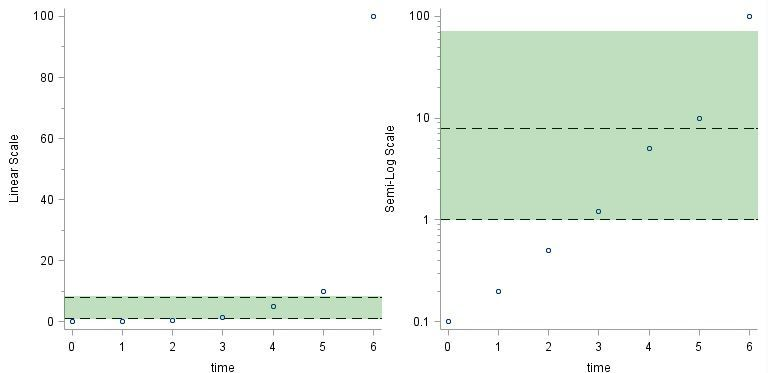## GTL: DRAWRECTANGLE wrong position on Log scale

I recently want to create an reference area from Y-axis 1 to 8 by using GTL. My codes worked for Linear Scale

(left side) but not for Log Scale (right side). It would be really appreciate if anyone knows how to correct it.```data have;
infile datalines missover;
input time value yreflower yrefupper ;
yrefrange = yrefupper - yreflower;
datalines;
0 0.1 1 8
1 0.2 1 8
2 0.5 1 8
3 1.2 1 8
4 5 1 8
5 10 1 8
6 100 1 8
;
run;

***** GTL *****;
proc template;
define statgraph Simple_Layout_Graphs/store=work.templat;
begingraph;
layout lattice / columns=2;

layout overlay /yaxisopts=(label='Linear Scale' linearopts=(viewmin=0  minorticks=true THRESHOLDMAX=1 THRESHOLDMIN=1)) WALLDISPLAY=NONE;
scatterplot x=time y=value;
referenceline y=yrefupper/ lineattrs=(color=black pattern=dash thickness=1);
referenceline y=yreflower/ lineattrs=(color=black pattern=dash thickness=1);
drawrectangle x=0 y=1 width=100 height=7 /display=(FILL) fillattrs=(color=green) transparency=0.75 anchor=bottomleft
xspace=wallpercent widthunit=percent yspace=datavalue heightunit=data;
endlayout;

layout overlay /yaxisopts=(label='Semi-Log Scale' type=log logopts=(base=10 tickintervalstyle=logexpand minorticks=true THRESHOLDMAX=1 THRESHOLDMIN=1)) WALLDISPLAY=NONE;
scatterplot x=time y=value;
referenceline y=yrefupper/ lineattrs=(color=black pattern=dash thickness=1);
referenceline y=yreflower/ lineattrs=(color=black pattern=dash thickness=1);
drawrectangle x=0 y=1 width=100 height=7 /display=(FILL) fillattrs=(color=green) transparency=0.75 anchor=bottomleft
xspace=wallpercent widthunit=percent yspace=datavalue heightunit=data;
endlayout;

endlayout;
endgraph;
end;

title;
footnote;

ods graphics / OUTPUTFMT=PNG noborder width=8in height=4in;

proc sgrender data=have template=Simple_Layout_Graphs;
run;```

1 ACCEPTED SOLUTION

Accepted Solutions

## Re: GTL: DRAWRECTANGLE wrong position on Log scale

Try height= 0.7 in the log section.

Since height=7 maps to 70 on the axis and your log base is 10 ...

4 REPLIES 4

## Re: GTL: DRAWRECTANGLE wrong position on Log scale

Try height= 0.7 in the log section.

Since height=7 maps to 70 on the axis and your log base is 10 ...

## Re: GTL: DRAWRECTANGLE wrong position on Log scale

Thank you. Height=0.7 works. But I do not understand why height needs change by 10 times smaller. I set heightunit=data, why it still be mapped to 70? I thought log base=10 is only for the axis scale display. Does it also affect the data mapping? I would be very happy if you have any further reference about this.

## Re: GTL: DRAWRECTANGLE wrong position on Log scale

Why, not exactly sure.

Looking through the documentation there are lots of interactions that seem to be possible between the axis definition and height and data units. I was actually wondering why there wasn't a Y2 value option to specify the second Y value of the rectangle instead of this sort of offset with "units".

Another approach might be use a bandplot instead of the rectangle but since that would likely require modification to the base data that may not be quite as flexible.